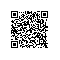# 文本自动分割算法

## 1.需求

• 中楼层/14层,东西,西直门南大街 3号院,1985年建,板楼
• 中楼层/23层,南北,通惠南路6号,2003年建,板楼
• 中楼层/12层,南北,通惠南路6号 1号院,2003年建,塔楼-##### 编译(发现内部的分隔符和模式)

def Compile(self,datas):

• 分隔符
• 相同的文本串(如上面的'楼层')
• 不同的文本串(如'14','12')
##### 分割(根据发现的模式分割)

def Split(self,text,splitgroup,isSameOut=True):

## 2.一种简单的思路

python
',' : [3,3,3]
'/' : [1,1,1]
' ' : [1,0,1]import re;

from asq.initiators import query

def GetVariance(data):
sum1 = 0.0
sum2 = 0.0
l = len(data);
for i in range(l):
sum1 += data[i]
sum2 += data[i] ** 2
mean = sum1 / l
var = sum2 / l - mean ** 2
return var;

def GetMaxSameCount(datas):
dic = {};
for t in datas:
if t in dic:
dic[t] += 1;
else:
dic[t] = 1;
if len(dic) == 0:
return 0;
maxkey, maxvalue = None, -1;
for key in dic:
if dic[key] > maxvalue:
maxvalue = dic[key];
maxkey = key;
return (maxkey, maxvalue);

class SplitType:
(ENTITY, SPLIT, SAMECONTENT, DIFFCONTENT) = range(4)

class SplitItem(object):
def __init__(self):
self.SplitType = None;
self.Name = None;
self.Value = None
self.Index = 0;
self.IsRepeat = False;

class SplitGroup(object):
def __init__(self):
self.SplitChars = {};  # dict,key:char, value:charmaxcount
self.SplitItems = [];

class Spliter(object):
def __init__(self):
self.MatchRatio = 0.8
self.ModeCheckRatio = 0.3;
self.MaxVariance = 3;
self.spliter2 = u' \r\n\t./_"\',;():|[]{}。：；'
self.spliter3 = re.compile(r'[a-zA-Z0-9\u4e00-\u9fa5\u3040-\u309f\u30a0-\u30ff]')
self.spliterdict = [self.spliter2, self.spliter3];

def GetCharCount(self, string, char):
count = 0;
for c in string:
if c == char:
count += 1;
return count;

def Compile(self, datas):
splititems = [];
splitchars = [];
maps = {};
datalen = len(datas);
for data in datas:
if data == None or data == '':
continue;
for splitchar in self.spliter2:
charcount = self.GetCharCount(data, splitchar)
if charcount == 0:
continue;
count = maps.get(splitchar, None);
if count == None:
maps[splitchar] = [charcount];
else:
maps[splitchar].append(charcount);
# select real splitchars
for text in maps:
map = maps[text];
if len(map) < datalen / 2:
continue
charcount = GetVariance(map);
maxkey, maxvalue = GetMaxSameCount(map);
if charcount < self.MaxVariance:
splitchars.append(text)
splitGroup = SplitGroup();
results = [];
modedict = [];
for data in datas:
splitResult = self.Split(data, splitchars);
results.append(splitResult);

qresults = query(results);
maxlen = qresults.max(lambda x: len(x));
samevalues = [];
for i in range(0, maxlen):
splititem = SplitItem();
splititem.Index = i;
values = [];
for splitResult in results:
if i < len(splitResult):
if splititem.SplitType == None and splitResult[i] in splitchars:
splititem.SplitType = SplitType.SPLIT;
splititem.Value = splitResult[i];
values.append(splitResult[i]);

if splititem.SplitType == None:
text, value = GetMaxSameCount(values)
if value > len(values) * self.MatchRatio:
splititem.SplitType = SplitType.SAMECONTENT;
splititem.Value = text;
if text in samevalues:
splititem.IsRepeat = True;
else:
samevalues.append(text);
else:
splititem.SplitType = SplitType.DIFFCONTENT;
splititems.append(splititem)
splitGroup.SplitChars = splitchars;
splitGroup.SplitItems = splititems;
# post process

return splitGroup;

def SplitWithGroup(self, text, splitgroup, isSameOut=True, issplitOut=False):
results = self.Split(text, splitgroup.SplitChars);
splitIndex = 0;
for r in results:
currp = splitgroup.SplitItems[splitIndex];
if r in splitgroup.SplitChars:
while splitgroup.SplitItems[splitIndex].Value != r:
splitIndex += 1;
if splitIndex == len(splitgroup.SplitItems):
return;
if issplitOut == False:
splitIndex += 1;
continue;
splitIndex += 1;
if currp.SplitType == SplitType.SAMECONTENT:
if isSameOut == False:
continue;
yield r;

def Split(self, data, splits):  # 连续的分隔符会被合并？
if data is None:
return None;
if len(splits) == 0:
return [data];
last = -1;
splititems = [];
l = len(data);
for i in range(0, l):
r = data[i];
if r not in splits:
continue;
else:
if i > 0 and i > last + 1:
splititems.append(data[last + 1:i]);
splititems.append(r);
last = i
if last + 1 < len(data):
splititems.append(data[last + 1:]);
return splititems;

if __name__ == '__main__':
sp = Spliter();
spgroups = sp.Compile(['中楼层/14层,东西,西直门南大街 3号院,1985年建,板楼'
, '中楼层/23层,南北,通惠南路6号,2003年建,板楼',
'中楼层/12层,南北,通惠南路6号 1号院,2003年建,塔楼'])
for r in sp.SplitWithGroup(u"低楼层/14层,东西,太阳宫中路太阳宫大厦,2003年建,板楼", spgroups):
print(r)

sp是分割器实例，对文本数组编译后获得了spgroups，这个数据结构存储了分割所需的信息。之后使用SplitWithGroup方法，即可对文本进行分割，返回的是一个生成器。该函数的一系列参数可以指定是否输出相同项：

## 3. 其他可能的方法

- 样本数量非常少
- 分隔符数量不一致
- 上文方法可能会带来严重的错误。

## 4.歧义性

LGA,MBA,12:03,15:04,165
MBA,LGA,18:30,22:40,174

## 5. 总结使用钉钉扫一扫加入圈子
+ 订阅